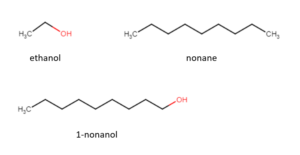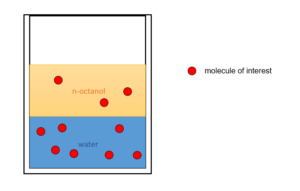# 53 Environmental Fate of Contaminants

Learning Objectives

By the end of this section, you should be able to:

Assess the physical properties of substances in order to determine their environmental fate if released

The environmental fate of contaminants describes where substances will go when released into the environment.

### Group Contribution Method

We may use our knowledge of chemical structures to predict the physical properties of the chemical substances.

Taking common chemical groups from a molecule, we can use the interactions from these groups to predict the properties the molecule will have based on parameters regressed from known molecules.

This is an example of how physical chemistry simulation and modeling can be used to predict important properties.

These properties can inform us of how a substance will act when dispersed in the environment.

Example: Group Contribution Method

The solubility of a substance is an important property for us to predict the way it migrates in the environment after release. Consider a simple situation where we have the following 3 compounds being released into a body of water:

Ethanol- a fuel commonly produced from biomass, also used as an industrial solvent for organic chemicals.

Nonane – a flammable liquid produced from petroleum.

1-nonanol – a compound commonly found in oils of citrus fruits and industrially used in manufacturing of artificial lemon oil.

The chemical structures of the 3 compounds are shown below:The -OH functional group is polar, which helps a compound to interact with and dissolve in water (a polar solvent). The long chain of alkanes are nonpolar and will decrease a compound’s solubility in water.

Using the group contribution analysis principle, ethanol will have the highest solubility in water due to a significant portion of its structure being polar (the -OH functional group). 1-nonanol will be less soluble owing to the larger carbon chain as opposed to ethanol. Nonane will be the least soluble as there are no polar groups in the molecule. There are group contribution correlations that allow us to calculate estimates of physical properties (solubility, boiling point, etc.) based on chemical structure, however, will not cover these methods in this course.

Experimental data for solubility of each compound is shown below, you’ll see this supports our rough analysis using the group contribution principles above:

Ethanol is miscible in water, which means it can dissolve with water of any proportions.

1-nonanol has a solubility of 0.13g/L in water.

Nonane has a solubility of 0.00002g/L in water.

We can conclude that if the three substances are released in a water body, ethanol will migrate much faster and will likely be more difficult to collect for treatment.

We can also use software to calculate physical properties. One such software is from the US EPA and is called EPI Suite – Estimation Program Interface Suite. It is only available to be run on windows and can be downloaded from https://www.epa.gov/tsca-screening-tools/epi-suitetm-estimation-program-interface.

Finding Chemical Properties Using EPI Suite
1. Input the CAS number of the molecule of interest in the “Input CAS #” field and click “Search CAS”. EPI Suite will find the structure based on the CAS Number. To find the CAS number, we can search for the compound on NIST, and the CAS number can be found on the general session on top of the page for the substance.

2. Click “Calculate” to calculate the physical properties.

3. The calculated properties will be shown in the pop-up window or on the bottom of the EPI suite window. We can scroll between tabs to see subsets of results.

## Predict the environmental fate of substances using physical property data

Example: Hazardous Materials & Contamination

Naphthalene, toluene, and methylene chloride are common hazardous materials used in the metal production industry. The following data is gathered from the EPI Suite software:

Property naphthalene toluene methylene chloride
Boiling point (°C) 217.9 110.6 40
$log_{10}$ of octanol/water partition coefficient 3.30 2.73 1.25
Water solubility(mg/L) 31 526 $1.3×10^4$
Henry’s law coefficient in water (atm·m3/mol) $4.40×10^{-4}$ $6.64×10^{-3}$ $3.25×10^{-3}$
$log_{10}$ of soil adsorption coefficient 3.189 2.369 1.337

Say a mixture of these three substances is accidentally released into the soil with some groundwater. Based on the physical property data:

a. Which substance is the most likely to be transported in the groundwater?

b. Which substance is the most likely to contaminate the soil?

Solution

a. Methylene chloride is the most likely to be transported in the water because of its much higher solubility compared to the other two substances.

b. Naphthalene is the most likely to contaminate the soil because it has the highest soil adsorption coefficient.

What is the octanol/water partition coefficient ($K_{ow}$)?

The octanol/water partition coefficient($K_{ow}$) can be measured by dissolving a substance in a container with n-octanol(a common non-polar solvent) and water(a common polar solvent). N-octanol is not soluble in water, so water and n-octanol will form separate phases. This allows us to measure the distribution of the third substance between water (a polar phase) and n-octanol (a non-polar phase).$K_{ow}$ is the ratio of the concentration of a substance dissolved in n-octanol and in water. The higher the $K_{ow}$, the less likely it is for the substance to dissolve in water. $K_{ow}$ is used in predicting the distribution of the substance when released into the environment if it is exposed to different solvents (such as water or oils).

$K_{ow}=\frac{\text{concentration of the substance in n-octanol}}{\text{concentration of the substance in water}}$

We often express the partition coefficient in the form of $log_{10}(K_{ow})$.

In general, the process of dissolving one substance in another is called absorption

Exercise: Using the Property of $K_{ow}$ to Select a Pollution Control Method

We will continue with the previous example with naphthalene, toluene, and methylene chloride. Their properties predicted by EPI Suite are shown below:

Property naphthalene toluene methylene chloride
Boiling point (°C) 217.9 110.6 40
$log_{10}$ of octanol/water partition coefficient 3.30 2.73 1.25
Water solubility(mg/L) 31 526 $1.3×10^4$
Henry’s law coefficient in water (atm·m3/mol) $4.40×10^{-4}$ $6.64×10^{-3}$ $3.25×10^{-3}$
$log_{10}$ of soil adsorption coefficient 3.189 2.369 1.337

a. Say each of these substances is present in a wastewater stream at a steel manufacturing plant in equal concentrations. We are to try to remove these substances by contacting them with a non-polar solvent that has similar properties to n-octanol (and leaving this for sufficient time). Which substance will have the lowest concentration in the wastewater stream after this contact?

b. Suppose the steel-manufacturing plant produces $1×10^{6}L$ of wastewater per day containing naphthalene at a concentration of $1.0×10^{-3}mol/L$. This wastewater is sent to a wastewater treatment plant, where the wastewater produced in one day is gathered and put in contract with 5000L of a non-polar solvent that has similar properties to n-octanol. What is the concentration of naphthalene in the water after this treatment? You can assume there is sufficient contact time for the solvent to absorb the naphthalene.

### Solution

a. Naphthalene will end up with the lowest concentration in the wastewater because it has the highest $K_{ow}$. This means out of the three substances naphthalene will have the largest proportion absorbed by the non-polar solvent.

b.  Step 1: Calculate the total moles of naphthalene:

$n=1.0×10^{-3}mol/L×1×10^{6}L=1000mol$

Step 2: Calculate the moles of naphthalene still dissolved in the water after the absorption process:

We can set the number of moles of naphthalene remaining in the water to be x mol, then the number of moles of naphthalene entering the non-polar solvent can be expressed as (1000-x)mol.

\begin{align*}
\frac{\text{concentration of the substance in nonpolar solvent}}{\text{concentration of the substance in water}}& = K_{ow} \\
\frac{\frac{1000-x}{5000}}{\frac{x}{1×10^{6}}}& = 10^{3.30} \\
\end{align*}

We can solve the equation using excel solver or a graphing calculator to find: $x=91.1 mol$.

Step 3: Calculate the final concentration of naphthalene:

$\frac{91.1mol}{1×10^{6}L}=9.11×10^{-5}mol/L$